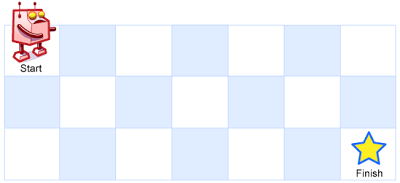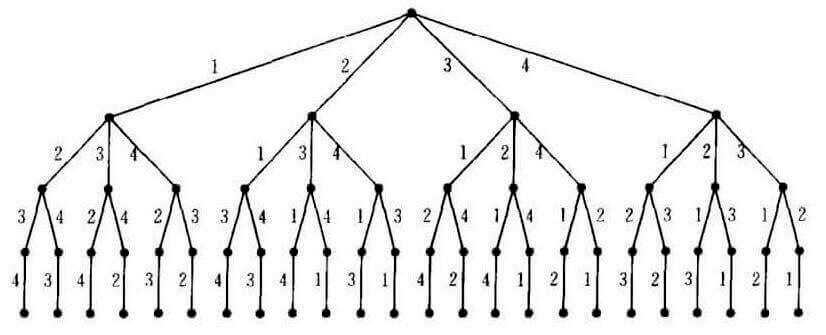LeetCode 63. Unique Paths II

Description

Unique Paths II

A robot is located at the top-left corner of a m x n grid (marked ‘Start’ in the diagram below).

The robot can only move either down or right at any point in time. The robot is trying to reach the bottom-right corner of the grid (marked ‘Finish’ in the diagram below).

Now consider if some obstacles are added to the grids. How many unique paths would there be?An obstacle and empty space is marked as 1 and 0 respectively in the grid.

Note: m and n will be at most 100.

Example 1:

Input:
[
[0,0,0],
[0,1,0],
[0,0,0]
]
Output: 2
Explanation:
There is one obstacle in the middle of the 3x3 grid above.
There are two ways to reach the bottom-right corner:
1. Right -> Right -> Down -> Down
2. Down -> Down -> Right -> Right

LeetCode 50. Pow(x, n)

Description

Pow(x, n)

Implement pow(x, n), which calculates x raised to the power n (x^n).

Example 1:

Input: 2.00000, 10
Output: 1024.00000

Example 2:

Input: 2.10000, 3
Output: 9.26100

Example 3:

Input: 2.00000, -2
Output: 0.25000
Explanation: 2^{-2} = {1/2}^2 = 1/4 = 0.25

Note:

• -100.0 < x < 100.0
• n is a 32-bit signed integer, within the range [−2^31, 2^31 − 1]

xn次方。

LeetCode 61. Rotate List

Description

Rotate List

Given a linked list, rotate the list to the right by k places, where k is non-negative.

Example 1:

Input: 1->2->3->4->5->NULL, k = 2
Output: 4->5->1->2->3->NULL
Explanation:
rotate 1 steps to the right: 5->1->2->3->4->NULL
rotate 2 steps to the right: 4->5->1->2->3->NULL

Example 2:

Input: 0->1->2->NULL, k = 4
Output: 2->0->1->NULL
Explanation:
rotate 1 steps to the right: 2->0->1->NULL
rotate 2 steps to the right: 1->2->0->NULL
rotate 3 steps to the right: 0->1->2->NULL
rotate 4 steps to the right: 2->0->1->NULL

LeetCode 60. Permutation Sequence

Description

Permutation Sequence

The set [1,2,3,...,n] contains a total of n! unique permutations.

By listing and labeling all of the permutations in order, we get the following sequence for n = 3:

1. "123"
2. "132"
3. "213"
4. "231"
5. "312"
6. "321"

Given n and k, return the k-th permutation sequence.

Note:

• Given n will be between 1 and 9 inclusive.
• Given k will be between 1 and n! inclusive.

Example 1:

Input: n = 3, k = 3
Output: "213"

Example 2:

Input: n = 4, k = 9
Output: "2314"LeetCode 59. Spiral Matrix II

Description

Spiral Matrix II

Given a positive integer n, generate a square matrix filled with elements from 1 to n^2 in spiral order.

Example:

Input: 3
Output:
[
[ 1, 2, 3 ],
[ 8, 9, 4 ],
[ 7, 6, 5 ]
]

LeetCode 58. Length of Last Word

Description

Length of Last Word

Given a string s consists of upper/lower-case alphabets and empty space characters ‘ ‘, return the length of last word in the string.

If the last word does not exist, return 0.

Note: A word is defined as a character sequence consists of non-space characters only.

Example:

Input: "Hello World"
Output: 5

LeetCode 57. Insert Interval

Description

Insert Interval

Given a set of non-overlapping intervals, insert a new interval into the intervals (merge if necessary).

You may assume that the intervals were initially sorted according to their start times.

Example 1:

Input: intervals = [[1,3],[6,9]], newInterval = [2,5]
Output: [[1,5],[6,9]]

Example 2:

Input: intervals = [[1,2],[3,5],[6,7],[8,10],[12,16]], newInterval = [4,8]
Output: [[1,2],[3,10],[12,16]]
Explanation: Because the new interval [4,8] overlaps with [3,5],[6,7],[8,10].

LeetCode 56. Merge Intervals

Description

Merge Intervals

Given a collection of intervals, merge all overlapping intervals.

Example 1:

Input: [[1,3],[2,6],[8,10],[15,18]]
Output: [[1,6],[8,10],[15,18]]
Explanation: Since intervals [1,3] and [2,6] overlaps, merge them into [1,6].

Example 2:

Input: [[1,4],[4,5]]
Output: [[1,5]]
Explanation: Intervals [1,4] and [4,5] are considerred overlapping.Programer

ChengDu·China